# First Law of Thermodynamics Notes | Study Mechanical Engineering SSC JE (Technical) - Mechanical Engineering

## Mechanical Engineering: First Law of Thermodynamics Notes | Study Mechanical Engineering SSC JE (Technical) - Mechanical Engineering

The document First Law of Thermodynamics Notes | Study Mechanical Engineering SSC JE (Technical) - Mechanical Engineering is a part of the Mechanical Engineering Course Mechanical Engineering SSC JE (Technical).
All you need of Mechanical Engineering at this link: Mechanical Engineering

FIRST LAW OF THERMODYNAMICS

• The First Law of Thermodynamics states that heat is a form of energy, and thermodynamic processes are therefore subject to the principle of conservation of energy. This means that heat energy cannot be created or destroyed. It can, however, be transferred from one location to another and converted to and from other forms of energy.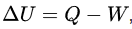where ΔU denotes the change in the internal energy  of a closed system

Q denotes the quantity of energy supplied to the system as heat, and

W denotes the amount of thermodynamic work done by the system on its surroundings.

System undergoing change of states

Qnet = ΔE +W
(Q+ Q3 – Q1) = ( ΔE +W2 +W3 - W 1- W4 )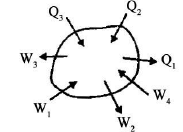• Energy is a point function and a property of the system. Energy is an extensive property while specific energy is an intensive property.
•  The internal energy depends only on temperature, The enthalpy also depends only on temperature for an ideal gas.

Heat transfer at constant volume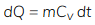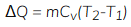Cv = speecific heat at constant volume

• Heat transfer at constant volume increases the internal energy of the system.

Heat transfer at constant pressure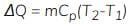Cp = Specific heat at constant pressure

•  Heat transfer at constant pressure increases the enthalpy of the system.

Heat transfer for an isolated system

dQ = 0, dw = 0 & dE = 0

• Energy of an isolated system is always constant.
• PMM-1 There can be no machine which would continuously supply mechanical work without absorbing some other form of energy simultaneously. Such a fictitions machine is called perpetual motion machine of first kind (PMM–1)
• The first law states the principle of conservation of energy.
• A PMM–1 is the impossible.
The document First Law of Thermodynamics Notes | Study Mechanical Engineering SSC JE (Technical) - Mechanical Engineering is a part of the Mechanical Engineering Course Mechanical Engineering SSC JE (Technical).
All you need of Mechanical Engineering at this link: Mechanical EngineeringUse Code STAYHOME200 and get INR 200 additional OFF

## Mechanical Engineering SSC JE (Technical)

6 videos|89 docs|55 tests

Track your progress, build streaks, highlight & save important lessons and more!

,

,

,

,

,

,

,

,

,

,

,

,

,

,

,

,

,

,

,

,

,

;